## Liquid to Gas Phase Transition

Vaporization of a sample of liquid is a phase transition from the liquid phase to the gas phase.

### Learning Objectives

Describe the process of vaporization.

### Key Takeaways

#### Key Points

• Evaporation is a phase transition from the liquid phase to the gas phase that occurs at temperatures below the boiling point at a given pressure.
• For molecules of a liquid to evaporate, they must be located near the surface, be moving in the proper direction, and have sufficient kinetic energy to overcome liquid-phase intermolecular forces.
• Boiling is a phase transition from the liquid phase to the gas phase that occurs at or above the boiling temperature.
• Boiling is the rapid vaporization of a liquid and occurs when a liquid is heated to its boiling point. A liquid’s boiling point is the temperature at which the vapor pressure of the liquid is equal to the pressure exerted on the liquid by the surrounding environment (air).

#### Key Terms

• Vaporization: Vaporization is a phase transition from the liquid phase to the gas phase.
• manometer: An instrument to measure pressure in a fluid, especially a double-legged liquid column gauge used to measure the difference in the pressures of two fluids.
• Boiling: Boiling is the rapid vaporization of a liquid and occurs when a liquid is heated to its boiling point, or the temperature at which the vapor pressure of the liquid is equal to the pressure exerted on the surface of the liquid by the surrounding atmospheric gas (air).
• Evaporation: A type of vaporization of a liquid that only occurs on the liquid’s surface.

### Phase Transition: Liquid to Gas

Vaporization of a sample of liquid is a phase transition from the liquid phase to the gas phase. There are two types of vaporization: evaporation and boiling.

• Evaporation occurs at temperatures below the boiling point, and occurs on the liquid’s surface. For molecules of a liquid to evaporate, they must be located near the surface, be moving in the proper direction, and have sufficient kinetic energy to overcome intermolecular forces present in the liquid phase.
• Boiling, by contrast, is a rapid vaporization that occurs at or above the boiling temperature and at or below the liquid’s surface.

### Vapor Pressure

In much the same way that tea spreads out from a tea bag once the bag is immersed in water, molecules that are confined within a phase will tend to spread themselves (and the thermal energy they carry with them) as widely as possible. This fundamental law of nature is manifested in what is called the “escaping tendency” of the molecules from the phase. The escaping tendency is of fundamental importance in understanding all chemical equilibria and transformations.

It is possible to observe the tendency of molecules to escape into the gas phase from a solid or liquid by placing the substance in a closed, evacuated container connected to a manometer for measuring gas pressure.

Vapor pressure: The vapor pressure is a direct measure of the escaping tendency of molecules from a condensed state of matter.

If this is done with water, the partial pressure of water Pw in the space above the liquid will initially be zero (step 1). Gradually, Pw will rise as molecules escape from the liquid phase and enter the vapor phase. At the same time, some of the vapor molecules will condense back into the liquid phase (step 2). Because this latter process is less favorable (at the particular temperature represented here), Pw continues to rise as more water vapor forms. Eventually a balance is reached between the two processes (step 3), and Pw stabilizes at a ﬁxed, or equilibrium, value Pvap, which depends on the substance and temperature. Pvap is known as the “equilibrium vapor pressure”, or simply as the “vapor pressure” of the liquid. The vapor pressure is a direct measure of the escaping tendency of molecules from a condensed state of matter.

### Boiling

The boiling point is the temperature at which the vapor pressure of the liquid is equal to the pressure exerted on the liquid by the surrounding environment (air). The escaping tendency of molecules from a phase always increases with temperature; therefore, the vapor pressure of a liquid will be greater at higher temperatures. The variation of vapor pressure with temperature is not linear. Because the typical pressure at the earth’s surface is 1 atm (760 torr), this is the vapor pressure that a liquid must equal in order for it to be at its normal boiling point.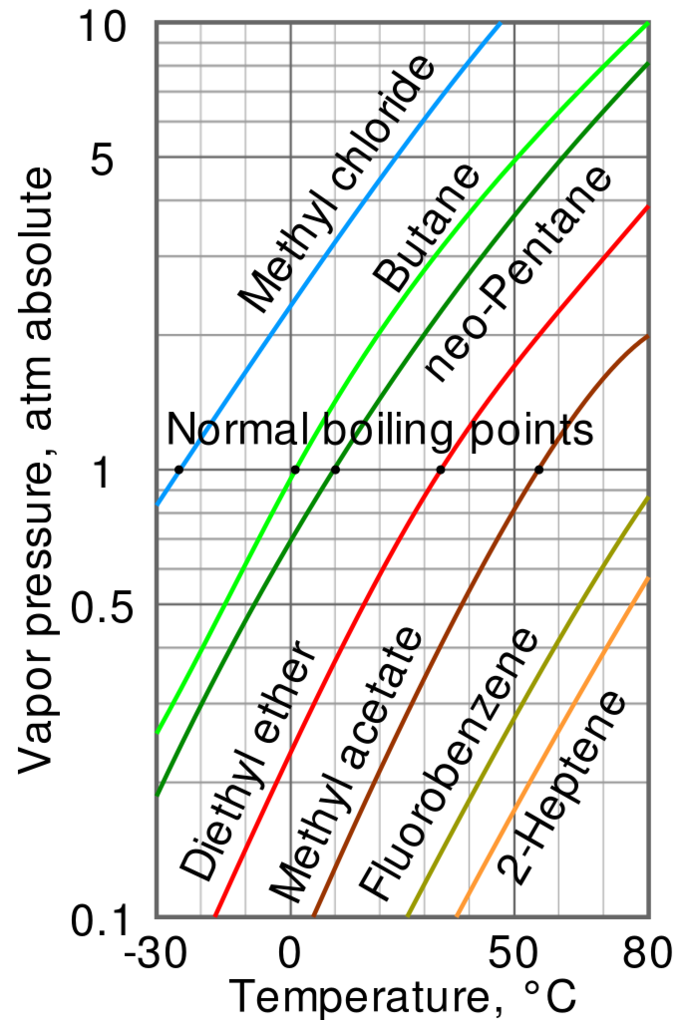Vapor pressure and temperature: The variation of vapor pressure with temperature is not linear. The intercepts of each curve with the horizontal line at 1 atm (i.e. 760 torr) indicate the normal boiling point of each liquid, ranging from -25 °C for methyl chloride to over 80 °C for fluorobenzene and 2-heptene.

The curve suggests that when the atmospheric pressure is lower than 1 atm (for instance, at higher altitudes), then the boiling point will occur at lower temperatures. This is because the liquid can be heated less in order for its vapor pressure to equal the atmospheric pressure. This has indeed been observed to be true.

### Related Phenomena Involving The Liquid Boundary Curvature

The vapor pressure of a liquid is determined by the attractive forces that act on the molecules at the surface of a liquid. In a very small drop, the liquid surface is curved in such a way that each molecule experiences fewer nearest-neighbor attractions than is the case for the bulk liquid. The outermost molecules of the liquid are bound to the droplet less tightly, and the drop has a larger vapor pressure than does the bulk liquid. If the vapor pressure of the drop is greater than the partial pressure of vapor in the gas phase, the drop will evaporate.

Interactive: Boiling Point: Non-polar molecules (gray) evaporate or boil more quickly than polar molecules (blue and red). Attractions between molecules are shown with dotted lines. Run the model, then heat the liquids. What does boiling look like at the molecular level?

A bubble is a hole in a liquid; molecules at the liquid boundary are curved inward, so they experience stronger nearest-neighbor attractions. As a consequence, the vapor pressure Pw of the liquid facing into a bubble is always less than that of the bulk liquid Pw at the same temperature. When the bulk liquid is at its normal boiling point (that is, when its vapor pressure is 1 atm), the pressure of the vapor within the bubble will be less than 1 atm, so the bubble will tend to collapse. Also, since the bubble is formed within the liquid, the hydrostatic pressure of the overlaying liquid will add to this eﬀect. For both of these reasons, a liquid will not boil until the temperature is raised slightly above the boiling point, a phenomenon known as superheating. Once the boiling begins, it will continue to do so at the liquid’s proper boiling point.

How does a liquid become a gas? – YouTube: This video looks at how vaporization and evaporation happens, and it addresses a big mistake that many people make when they deal with the liquid gas phase transition.

## Supercritical Fluids

A supercritical fluid is a substance at a temperature and pressure above its critical point, where distinct liquid and gas phases do not exist.

### Learning Objectives

Discuss the properties of supercritical fluids.

### Key Takeaways

#### Key Points

• Supercritical fluids have properties between those of a gas and a liquid.
• A supercritical fluid can effuse through solids like a gas and dissolve materials like a liquid.
• All supercritical fluids are completely miscible with each other, so for a mixture a single phase can be guaranteed, if the critical point of the mixture is exceeded.

#### Key Terms

• supercritical fluid: Any substance at a temperature and pressure above its critical point, where distinct liquid and gas phases do not exist.
• critical temperature: The temperature beyond which no phase boundaries exist for a given substance.
• critical point: Also known as a critical state, this point occurs under conditions (such as specific values of temperature, pressure, or composition) at which no phase boundaries exist.
• critical pressure: The pressure beyond which no phase boundaries exist for a given substance.

### Properties of Supercritical Fluids

A supercritical fluid is any substance at a temperature and pressure above its critical point, where distinct liquid and gas phases do not exist. This can be rationalized by thinking that at high enough temperatures (above the critical temperature ) the kinetic energy of the molecules is high enough to overcome any intermolecular forces that would condense the sample into the liquid phase. On the other hand, high enough pressures (above the critical pressure ) would not allow a sample to stay in the pure gaseous state. Therefore, a balance between these two tendencies is achieved and the substance exists in a state between a gas and a liquid.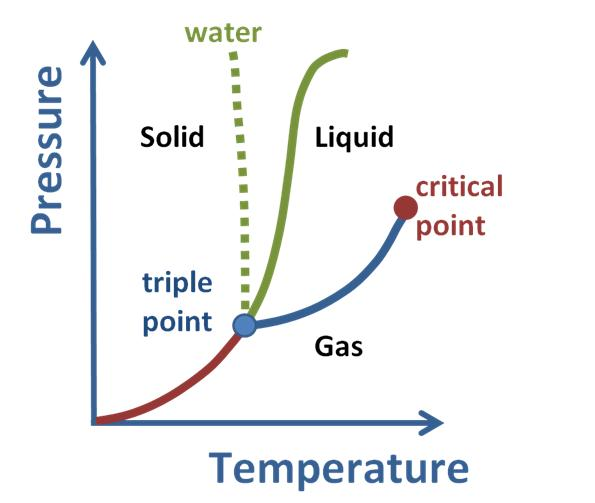Phase Diagram for a Substance: The figure highlights the critical point, above which (in either temperature or pressure) the substance does not exist in either the liquid or gas phase. Under those conditions it is called a “supercritical fluid,” and has properties between those of a liquid and a gas.

It can effuse through solids (like a gas), and dissolve materials (like a liquid). In addition, close to the critical point, small changes in pressure or temperature result in large changes in density, allowing many properties of a supercritical fluid to be “fine-tuned. ” Supercritical fluids are suitable as a substitute for organic solvents in a range of industrial and laboratory processes. Carbon dioxide and water are the most commonly used supercritical fluids, as they are used for decaffeination and power generation, respectively.

In general terms, supercritical fluids have properties between those of a gas and a liquid. The critical properties of some substances used as solvents and as supercritical fluids are shown in Table 1. Table 2 shows density, diffusivity, and viscosity for typical liquids, gases, and supercritical fluids.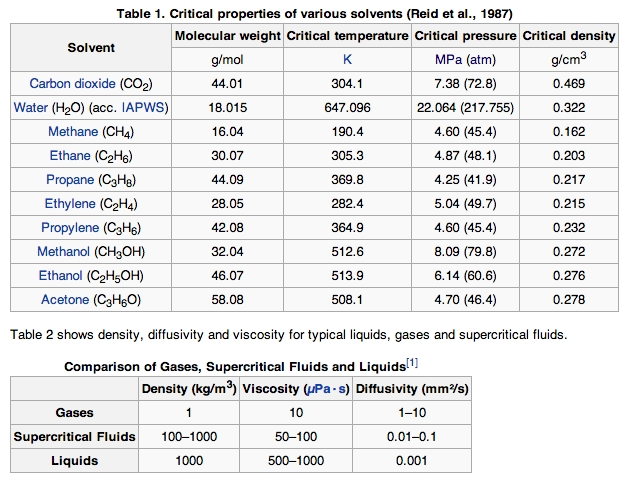Critical Properties of Various Solvents: Supercritical fluids have properties between those of a gas and a liquid.

In addition, there is no surface tension in a supercritical fluid, as there is no liquid to gas phase boundary. By changing the pressure and temperature of the fluid, the properties can be “tuned” to be more liquid- or gas-like. One of the most important properties of supercritical fluids is their ability to act as solvents. Solubility in a supercritical fluid tends to increase with the density of the fluid (at constant temperature). Since density increases with pressure, solubility tends to increase with pressure.

The relationship with temperature is a little more complicated. At constant density, solubility will increase with temperature. However, close to the critical point, the density can drop sharply with a slight increase in temperature. Therefore, close to the critical temperature, solubility often drops with increasing temperature, then rises again.

All supercritical fluids are completely miscible with each other; therefore a single phase for a mixture can be guaranteed if the critical point is exceeded. The critical point of a binary mixture can be estimated as the arithmetic mean of the critical temperatures and pressures of the two components,

Tc(mix) = (mole fraction of A) x Tc(A) + (mole fraction of B) x Tc(B)

For greater accuracy, the critical point can be calculated using equations of state, such as the Peng Robinson or group contribution methods. Other properties, such as density, can also be calculated using equations of state.

### Case Study: Carbon Dioxide

In the pressure-temperature phase diagram of CO2, the boiling separates the gas and liquid region and ends in the critical point, where the liquid and gas phases disappear to become a single supercritical phase. At well below the critical temperature, (e.g., 280 K), as the pressure increases, the gas compresses and eventually (at just over 40 bar) condenses into a much denser liquid, resulting in the discontinuity in the line (vertical dotted line). The system consists of 2 phases in equilibrium, a dense liquid and a low density gas.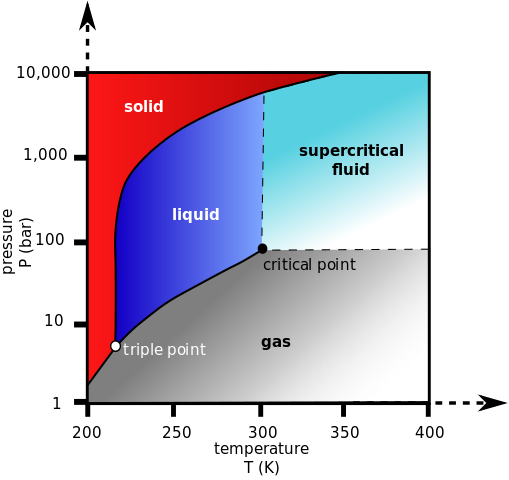Phase Diagram for Carbon Dioxide: This diagram indicates the supercritical fluid region of CO2.

As the critical temperature is approached (300 K), the density of the gas at equilibrium becomes denser, and that of the liquid becomes lower. At the critical point, (304.1 K and 7.38 MPa) there is no difference in density, and the two phases become one fluid phase. Thus, above the critical temperature a gas cannot be liquified by pressure. At slightly above the critical temperature (310 K), in the vicinity of the critical pressure, the line is almost vertical. A small increase in pressure causes a large increase in the density of the supercritical phase. Many other physical properties also show large gradients with pressure near the critical point, such as viscosity, the relative permittivity, and the solvent strength, which are all closely related to the density.

A close look at supercritical carbon dioxide: A pressure vessel made of aluminum and acrylic is filled with pieces of dry ice. The dry ice melts under high pressure, and forms a liquid and gas phase. When the vessel is heated, the CO2 becomes supercritical — meaning the liquid and gas phases merge together into a new phase that has properties of a gas, but the density of a liquid. Supercritical CO2 is a good solvent, and is used for decaffeinating coffee, dry cleaning clothes, and other situations where avoiding a hydrocarbon solvent is desirable for environmental or health reasons.

## Liquid to Solid Phase Transition

Freezing is a phase transition in which a liquid turns into a solid when its temperature is lowered to its freezing point.

### Learning Objectives

Discuss the process of freezing.

### Key Takeaways

#### Key Points

• For most substances, the melting and freezing points are the same temperature; however, certain substances possess different solid – liquid transition temperatures.
• Most liquids freeze by crystallization, the formation of a crystalline solid from the uniform liquid.
• Freezing is almost always an exothermic process, meaning that as liquid changes into solid, heat is released.
• The energy released upon freezing, known as the enthalpy of fusion, is a latent heat, and is exactly the same as the energy required to melt the same amount of the solid.

#### Key Terms

• Freezing: Freezing or solidification is a phase transition in which a liquid turns into a solid when its temperature is lowered to its freezing point.
• Nucleation: In the context of freezing, nucleation is the localized budding of a crystalline solid structure.

Freezing, or solidification, is a phase transition in which a liquid turns into a solid when its temperature is lowered to or below its freezing point. All known liquids, except helium, freeze when the temperature is low enough. (Liquid helium remains a liquid at atmospheric pressure even at absolute zero, and can be solidified only under higher pressure.)

For most substances, the melting and freezing points are the same temperature; however, certain substances possess different solid-liquid transition temperatures. For example, agar displays a hysteresis in its melting and freezing temperatures: it melts at 85 °C (185 °F) and solidifies between 31 °C and 40 °C (89.6 °F to 104 °F).

Most liquids freeze by crystallization, the formation of a crystalline solid from the uniform liquid.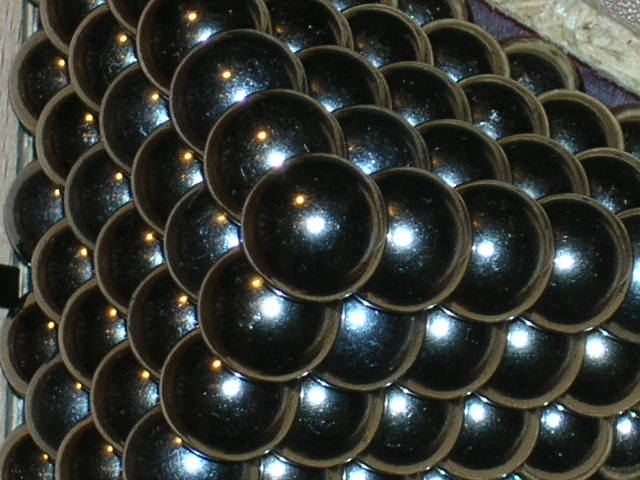Crystalline Solid: Model of closely packed atoms within a crystalline solid.

### Nucleation

This is a first-order thermodynamic phase transition, which means that as long as solid and liquid coexist, the equilibrium temperature of the system remains constant and equal to the melting point. Crystallization consists of two major events: nucleation and crystal growth. Nucleation is the step in which the molecules start to gather into clusters (on the scale of nanometers), arranging themselves in the periodic pattern that defines the crystal structure. The crystal growth is the subsequent growth of the nuclei that succeed in achieving and surpassing the critical cluster size.Nucleation Leads to Crystal Formation: When sugar is supersaturated in water, nucleation will occur, allowing sugar molecules to stick together and form large crystal structures.

Crystallization of pure liquids usually begins at a lower temperature than the melting point, due to the high activation energy of homogeneous nucleation. The creation of a nucleus implies the formation of an interface at the boundaries of the new phase. Some energy is expended to form this interface, based on the surface energy of each phase. If a hypothetical nucleus is too small, the energy that would be released by forming its volume is not enough to create its surface, and nucleation does not proceed. Freezing does not start until the temperature is low enough to provide enough energy to form stable nuclei.

In the presence of irregularities on the surface of the containing vessel, solid or gaseous impurities, pre-formed solid crystals, or other nucleators, heterogeneous nucleation may occur. Heterogeneous nucleation is when nucleation occurs on a surface that the substance is in contact with.

The melting point of water at one atmosphere of pressure is very close to 0 °C (32 °F, 273.15 K), and in the presence of nucleating substances the freezing point of water is close to the melting point. However, in the absence of nucleators water can supercool to -40 °C (-40 °F, 233 K) before freezing. Under high pressure (2,000 atmospheres), water will supercool to as low as -70 °C (-94 °F, 203 K) before freezing.

### Freezing is Accompanied by Release of Heat

Freezing is almost always an exothermic process, meaning that as liquid changes into solid, heat is released. This may seem counterintuitive, since the temperature of the material does not rise during freezing (except if the liquid is supercooled). But heat must be continually removed from the freezing liquid, or the freezing process will stop. The energy released upon freezing, known as the enthalpy of fusion, is a latent heat and is exactly the same as the energy required to melt the same amount of the solid.

Interactive: Phase Change: Matter exists as solids, liquids and gases, and can change state between these. The model shows a liquid material on the left (small atoms). The amount of heat energy is shown by kinetic energy (KE) shading, with deeper shades of red representing more energetic atoms. On the right side of the barrier is a solid material (large atoms). Run the model. How much energy is able to penetrate the barrier? Remove the barrier. How quickly do the more energetic atoms melt the solid?

## Solid to Gas Phase Transition

Sublimation is the phase transition from the solid to the gaseous phase, without passing through an intermediate liquid phase.

### Learning Objectives

Discuss the process of sublimation.

### Key Takeaways

#### Key Points

• Sublimation is an endothermic phase transition in which a solid evaporates to a gas.
• Solids that sublimate have such high vapor pressures that heating leads to a substantial vaporization even before the melting point is reached.
• The enthalpy of sublimation (also called heat of sublimation) can be calculated as the sum of the enthalpy of fusion and the enthalpy of vaporization.

#### Key Terms

• sublimation: The process of transformation directly from the solid to the gaseous phase, without passing through an intermediate liquid phase.
• Triple point: In thermodynamics, the triple point of a substance is the temperature and pressure at which the three phases (gas, liquid, and solid) coexist in thermodynamic equilibrium.
• deposition: A phase transition in which a gas is converted to solid, without passing though an intermediate liquid phase. It is the reverse process of sublimation.

### Phase Transition: Solid to Gas

Sublimation is the process of transformation directly from the solid phase to the gaseous phase, without passing through an intermediate liquid phase. It is an endothermic phase transition that occurs at temperatures and pressures below a substance ‘s triple point (the temperature and pressure at which all three phases coexist) in its phase diagram.

At a given temperature, most chemical compounds and elements can possess one of the three different states of matter at different pressures. In these cases, the transition from the solid to the gaseous state requires an intermediate liquid state. But at temperatures below that of the triple point, a decrease in pressure will result in a phase transition directly from the solid to the gaseous. Also, at pressures below the triple point pressure, an increase in temperature will result in a solid being converted to gas without passing through the liquid region.Phase Diagram of a Pure Substance: Notice the triple point of the substance. At temperatures and pressures below those of the triple point, a phase change between the solid and gas phases can take place.

For some substances, such as carbon and arsenic, sublimation is much easier than evaporation. This is because the pressure of their triple point is very high and it is difficult to obtain them as liquids. The solid has such high vapor pressures that heating leads to a substantial amount of direct vaporization even before the melting point is reached.

The process of sublimation requires additional energy and is therefore an endothermic change. The enthalpy of sublimation (also called heat of sublimation) can be calculated as the sum of the enthalpy of fusion and the enthalpy of vaporization.

The reverse process of sublimation is deposition (i.e., gas to solid). For example, solid iodine, I2, is easily sublimed at temperatures around 100°C. Even ice has a measurable vapor pressure near its freezing point, as evidenced by the tendency of snow to evaporate in cold dry weather. There are other solids whose vapor pressure overtakes that of the liquid before melting can occur. Such substances sublime; a common example is solid carbon dioxide (dry ice) at 1 atm of atmospheric pressure.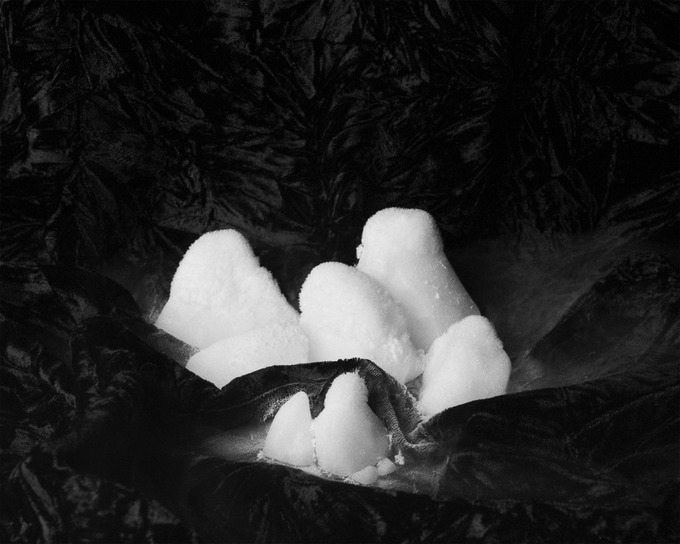Dry Ice: Solid carbon dioxide (known as “dry ice”) sublimes into the air.

## Heating Curve for Water

Water transitions from ice to liquid to water vapor as heat is added to it.

### Learning Objectives

Discuss the heating curve for water.

### Key Takeaways

#### Key Points

• A heating curve graphically represents the phase transitions that a substance undergoes as heat is added to it.
• The plateaus on the curve mark the phase changes. The temperature remains constant during these phase transitions.
• Water has a high boiling point because of the strong hydrogen bonds between the water molecules; it is both a strong hydrogen bond donor and acceptor.
• The first change of phase is melting, during which the temperature stays the same while water melts. The second change of phase is boiling, as the temperature stays the same during the transition to gas.

#### Key Terms

• hydrogen bond: A strong intermolecular bond in which a hydrogen atom in one molecule is attracted to a highly electronegative atom (usually nitrogen or oxygen) in a different molecule.
• specific heat capacity: The amount of heat needed to raise the temperature of 1 g of a substance by 1 degree Celsius.

Like many substances, water can exist in different phases of matter: liquid, solid, and gas. A heating curve shows how the temperature changes as a substance is heated up at a constant rate.

### Drawing a Heating Curve

Temperature is plotted on the y-axis, while the x-axis represents the heat that has been added. A constant rate of heating is assumed, so that one can also think of the x-axis as the amount of time that goes by as a substance is heated. There are two main observations on the measured curve:

• regions where the temperature increases as heat is added
• plateaus where the temperature stays constant.

It is at those plateaus that a phase change occurs.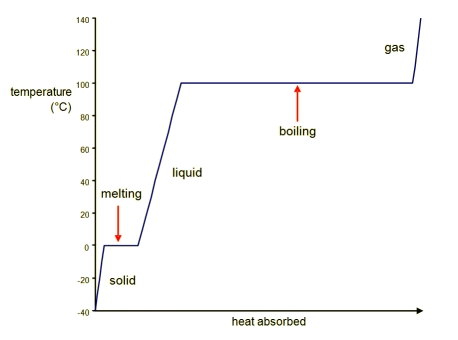Heating Curve of Water: The phase transitions of water.

### Analysis of a Heating Curve

Looking from left to right on the graph, there are five distinct parts to the heating curve:

1. Solid ice is heated and the temperature increases until the normal freezing/melting point of zero degrees Celsius is reached. The amount of heat added, q, can be computed by: $\text{q}=\text{m}\cdot \text{C}_{\text{H}_2\text{O}(\text{s})}\cdot \Delta \text{T}$, where m is the mass of the sample of water, C is the specific heat capacity of solid water, or ice, and $\Delta \text{T}$ is the change in temperature during the process.
2. The first phase change is melting; as a substance melts, the temperature stays the same. For water, this occurs at 0o C. The above equation (described in part 1 of the curve) cannot be used for this part of the curve because the change in temperature is zero! Instead, use the heat of fusion ($\Delta \text{H}_{\text{fusion}}$ ) to calculate how much heat was involved in that process: $\text{q}=\text{m}\cdot \Delta \text{H}_{\text{fusion}}$, where m is the mass of the sample of water.
3. After all of the solid substance has melted into liquid, the temperature of the liquid begins to increase as heat is absorbed. It is then possible to calculate the heat absorbed by: $\text{q}=\text{m}\cdot \text{C}_{\text{H}_2\text{O}(\text{l})}\cdot \Delta \text{T}$. Note that the specific heat capacity of liquid water is different than that of ice.
4. The liquid will begin to boil when enough heat has been absorbed by the solution that the temperature reaches the boiling point, where again, the temperature remains constant until all of the liquid has become gaseous water. At the atmospheric pressure of 1 atm, this phase transition occurs at 100o C (the normal boiling point of water). Liquid water becomes water vapor or steam when it enters the gaseous phase. Use the heat of vaporization ($\Delta \text{H}_{\text{vap}}$ ) to calculate how much heat was absorbed in this process: $\text{q}=\text{m}\cdot \text{C}_{\text{H}_2\text{O}(\text{g})}\cdot \Delta \text{T}$, where m is the mass of the sample of water.
5. After all of the liquid has been converted to gas, the temperature will continue to increase as heat as added. Again, the heat added that results in a certain change temperature is given by: $\text{q}=\text{m}\cdot \text{C}_{\text{H}_2\text{O}(\text{g})}\cdot \Delta T$. Note that the specific heat capacity of gaseous water is different than that of ice or liquid water.
6. Water has a high boiling point because of the presence of extensive hydrogen bonding interactions between the water molecules in the liquid phase (water is both a strong hydrogen bond donor and acceptor). When heat is first applied to water, it must break the intermolecular hydrogen bonds within the sample. After breaking the bonds, heat is then absorbed and converted to increased kinetic energy of the molecules in order to vaporize them.